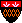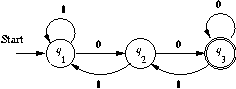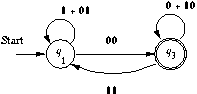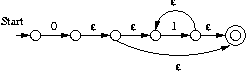Introduction to Automata Theory, Languages, and Computation

## Solutions for Chapter 3

Solutions for Section 3.1

## Solutions for Section 3.1

### Exercise 3.1.1(a)

The simplest approach is to consider those strings in which the first a precedes the first b separately from those where the opposite occurs. The expression: c*a(a+c)*b(a+b+c)* + c*b(b+c)*a(a+b+c)*

### Exercise 3.1.2(a)

(Revised 9/5/05) The trick is to start by writing an expression for the set of strings that have no two adjacent 1's. Here is one such expression: (10+0)*(ε+1)

To see why this expression works, the first part consists of all strings in which every 1 is followed by a 0. To that, we have only to add the possibility that there is a 1 at the end, which will not be followed by a 0. That is the job of (ε+1).

Now, we can rethink the question as asking for strings that have a prefix with no adjacent 1's followed by a suffix with no adjacent 0's. The former is the expression we developed, and the latter is the same expression, with 0 and 1 interchanged. Thus, a solution to this problem is (10+0)*(ε+1)(01+1)*(ε+0). Note that the ε+1 term in the middle is actually unnecessary, as a 1 matching that factor can be obtained from the (01+1)* factor instead.

### Exercise 3.1.4(a)

This expression is another way to write ``no adjacent 1's.'' You should compare it with the different-looking expression we developed in the solution to Exercise 3.1.2(a). The argument for why it works is similar. (00*1)* says every 1 is preceded by at least one 0. 0* at the end allows 0's after the final 1, and (ε+1) at the beginning allows an initial 1, which must be either the only symbol of the string or followed by a 0.

### Exercise 3.1.5

The language of the regular expression ε. Note that ε* denotes the language of strings consisting of any number of empty strings, concatenated, but that is just the set containing the empty string.

## Solutions for Section 3.2

### Exercise 3.2.1

Part (a): The following are all R0 expressions; we list only the subscripts. R11 = ε+1; R12 = 0; R13 = phi; R21 = 1; R22 = ε; R23 = 0; R31 = phi; R32 = 1; R33 = ε+0.

Part (b): Here all expression names are R(1); we again list only the subscripts. R11 = 1*; R12 = 1*0; R13 = phi; R21 = 11*; R22 = ε+11*0; R23 = 0; R31 = phi; R32 = 1; R33 = ε+0.

Part (e): Here is the transition diagram:If we eliminate state q2 we get:Applying the formula in the text, the expression for the ways to get from q1 to q3 is: [1 + 01 + 00(0+10)*11]*00(0+10)*

### Exercise 3.2.4(a)### Exercise 3.2.6(a)

(Revised 1/16/02) LL* or L+.

### Exercise 3.2.6(b)

The set of suffixes of strings in L.

### Exercise 3.2.8

Let R(k)ijm be the number of paths from state i to state j of length m that go through no state numbered higher than k. We can compute these numbers, for all states i and j, and for m no greater than n, by induction on k.

Basis: R0ij1 is the number of arcs (or more precisely, arc labels) from state i to state j. R0ii0 = 1, and all other R0ijm's are 0.

Induction: R(k)ijm is the sum of R(k-1)ijm and the sum over all lists (p1,p2,...,pr) of positive integers that sum to m, of R(k-1)ikp1 * R(k-1)kkp2 *R(k-1)kkp3 *...* R(k-1)kkp(r-1) * R(k-1)kjpr. Note r must be at least 2.

The answer is the sum of R(k)1jn, where k is the number of states, 1 is the start state, and j is any accepting state.

## Solutions for Section 3.4

### Exercise 3.4.1(a)

Replace R by {a} and S by {b}. Then the left and right sides become {a} union {b} = {b} union {a}. That is, {a,b} = {b,a}. Since order is irrelevant in sets, both languages are the same: the language consisting of the strings a and b.

### Exercise 3.4.1(f)

Replace R by {a}. The right side becomes {a}*, that is, all strings of a's, including the empty string. The left side is ({a}*)*, that is, all strings consisting of the concatenation of strings of a's. But that is just the set of strings of a's, and is therefore equal to the right side.

### Exercise 3.4.2(a)

Not the same. Replace R by {a} and S by {b}. The left side becomes all strings of a's and b's (mixed), while the right side consists only of strings of a's (alone) and strings of b's (alone). A string like ab is in the language of the left side but not the right.

### Exercise 3.4.2(c)

Also not the same. Replace R by {a} and S by {b}. The right side consists of all strings composed of zero or more occurrences of strings of the form a...ab, that is, one or more a's ended by one b. However, every string in the language of the left side has to end in ab. Thus, for instance, ε is in the language on the right, but not on the left.